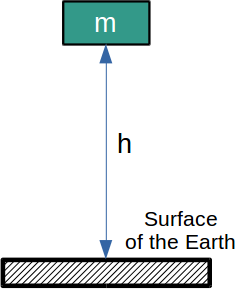# How to calculate potential energy

### Definition

Potential energy is the capacity of a body or object to do work due to its position. In the general case, potential energy can be defined as the capacity of an object to do work due to its position or configuration.

Potential energy appears due to external forces which act upon object. These forces can be gravitational, magnetic or electric.

For example, any object or body with a mass and a certain height from the Earth’s surface has a potential energy. If the object or body is not raised above the ground, it will have zero height therefore zero potential energy. The higher the distance from the surface of the Earth, the higher the potential energy.Image: Potential energy

Since all objects which are at a particular distance from the surface of the Earth are attracted towards it due to gravitational force, the potential energy is also called gravitational potential energy.

Go back

### Formula

To calculate potential energy multiply the mass of the body (object) with the gravitational acceleration and the height from the surface of the Earth. The formula (equation) to calculate potential energy is :

Ep = m · g · h
(1)

where:

• Ep [J] – potential energy
• m [kg] – mass
• g [m/s2] – gravitational acceleration
• h [m] – height (measured from the surface of the Earth)

The unit of measurement of potential energy is joule [J].

Go back

### Rock example

Calculate the potential energy of a rock of mass 500 g, held at a height of 2 m above ground.

Step 1. Convert the mass of the rock from [g] to [kg], by dividing the [g] value to 1000:

m = 500 / 1000 = 0.5 kg

Step 2. Calculate the potential energy Ep [J] of the rock using equation (1):

Ep = m · g · h = 0.5 · 9.81 · 2 = 9.81 J

Go back

### Bowling ball example

Calculate the potential energy of a bowling ball of mass 7 kg, held at 50 cm above ground.

Step 1. Convert the height from [cm] to [m], by dividing the [cm] to 100:

m = 50 / 100 = 0.5 m

Step 2. Calculate the potential energy Ep [J] of the bowling ball using equation (1):

Ep = m · g · h = 7 · 9.81 · 0.5 = 34.335 J

Go back

### Calculator

The potential energy calculator allows you to calculate the potential energy of a body (object) with a given mass and height above the ground. You need to enter the mass and height parameters and choose the desired unit of measurement.

The default unit of measurement for energy is Joule. If you want the result displayed in another unit, use the dropt down list to choose and click the CALCULATE button again.

Go back

 David Halliday, Robert Resnick, Jearl Walker, Fundamentals of Physics, 7th edition, John Wiley & Sons, 2004.
 Benjamin Crowell, Light and Matter – Physics, 2007.
 Raymond A. Serway and John W. Jr. Jewett, Physics for Scientists and Engineers, 6th edition, Brooks/Cole Publishing Co.,2004
 Jiansong Li, Jiyun Zhao, and Xiaochun Zhang, A Novel Energy Recovery System Integrating Flywheel and Flow Regeneration for a Hydraulic Excavator Boom System, Energies 2020.
 Leo H. Holthuijsen, Waves in oceanic and coastal waters, Cambridge University Press, 2007.
 Kira Grogg, Harvesting the Wind: The Physics of Wind Turbines, Carleton College, 2005.